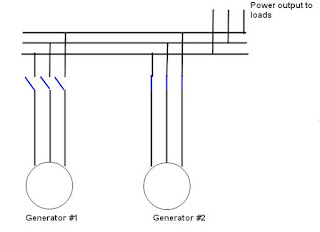# Synchronization of AC power sources

Synchronization is a process involved in connecting two AC power supplies. AC power sources
such as transformers and generators need to be operated in parallel. This may be required
for greater reliability and capacity sharing.

In the picture, the incoming power source, a generator(#1) is being synchronized with a busbar which is already to a generator(#2) which is running.For synchronizing three conditions need to be satisfied
1. Equal Voltages
By Equal voltages, we mean that the rms value of the voltages should be equal.

2. Equal frequencies
Equal frequencies implies that the two sources should have equal frequencies. A 50Hz source
can only be synchronized with another source whose frequency is also 50 Hz.

3. Same Phase Sequence
The sources should have similar phase sequence i.e. RYB and RYB or RBY and RBY.4. Same Phase angle
The vectors of the two sources should have the same phase angle.

It is possible to bring the frequency, phase angle and voltage within synchronizing limits by adjusting the speed and voltage of the generator.

The circuit breaker connecting the two power sources can be closed when all these conditions are satisfied. The drawing shows vector diagrams of two three phase voltages whose phase sequences, phase angles, voltages and frequencies are equal

Special equipment and checks are used to ensure that the above conditions are satisfied.